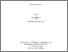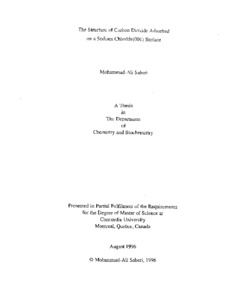Title:

# The structure of carbon dioxide adsorbed on a sodium chloride (001) surface

Saberi, Mohammad-Ali (1996) The structure of carbon dioxide adsorbed on a sodium chloride (001) surface. Masters thesis, Concordia University.Preview
Text (application/pdf)
MM18435.pdf
2MB

## Abstract

Two sets of parameters and potential energy surfaces for a single CO$\sb2$ molecule adsorbed on the (001) face of a uniform semi-infinite crystal of NaCl were developed and tested using the Steepest Descent method. When the CO$\sb2$ molecule attains the absolute minimum of the potential energy it was found, for both parameter sets, to lie parallel to the surface at height z = 2.5 A above the connection line of two adjacent Na$\sp+$ ions (along the $\langle 110\rangle$ direction) at 1.994 A from Na$\sp+$ (C to Na$\sp+$); i.e. the carbon atom is directly above the midpoint between two sodium ions. The values of the potential energy were found to be E$\sb\min = {-}7.643$ kcal/mol and E$\sb\min = {-}8.000$ kcal/mol for parameters set I and set II respectively. Saddle points in the potential energy surfaces were found to exist above the connection line between Na$\sp+$ and Cl$\sp-$ (the $\langle 100\rangle$ direction). The position of this point correspond to a lateral sodium to carbon distance of 0.92 A and a carbon height of z = 2.95A above the surface for parameter set I. At this position the molecule is tilted by an angle of $35\sp\circ$ from surface $(55\sp\circ$ from the surface normal). The potential energy at this point was found to be E$\sb{\rm sad} = {-}5.723$ kcal/mol which yielded an estimated diffusion barrier energy of E$\sb{\rm diff} = 1.918$ kcal/mol. The parameter set II was examined the similar results was obtained. The single molecule surface potential which was developed was then used within the Metropolis Monte Carlo method to examine monolayer, bilayer and trilayer configurations of CO$\sb2$ on an NaCl(001) surface. (Abstract shortened by UMI.)

Divisions: Concordia University > Faculty of Arts and Science > Chemistry and Biochemistry Thesis (Masters) Saberi, Mohammad-Ali xi, 101 leaves : ill. ; 29 cm. Concordia University M.Sc. Chemistry 1996 Jack, David B QD 181 C1S23 1996 158 Concordia University Library 27 Aug 2009 17:10 13 Jul 2020 19:45 https://concordiauniversity.on.worldcat....
All items in Spectrum are protected by copyright, with all rights reserved. The use of items is governed by Spectrum's terms of access.

Repository Staff Only: item control pageResearch related to the current document (at the CORE website)
Back to top